# 含参模糊决策蕴涵

1(山西大学计算机与信息技术学院 太原 030006)

2(计算智能与中文信息处理教育部重点实验室(山西大学) 太原 030006)

(chai_yanhui@163.com)

## 1 模糊逻辑

1) (L,∧,∨,0,1)是以0和1为最小元和最大元的完备格；

2) (L,⊗,1)为交换幺半群，即⊗满足交换律和结合律，且有∀aL,a⊗1=1⊗a=a

3) ⊗，→为伴随对，满足伴随条件abc当且仅当abc，其中a,b,cL.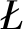ukasiewicz：

ab=max(a+b-1,0)，
ab=min(1-a+b,1).

Godel：

ab=min(a,b)，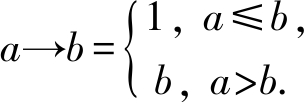Goguen：

ab=a·b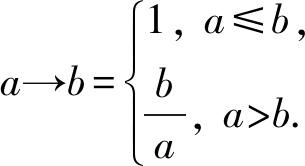1) 1*=1, a*a

2) (ab)*a*b*

3) a**=a*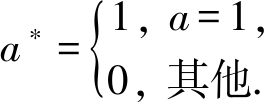1) abab=1；

2) abcbac

3) (ab)→c=(a→(bc))；

4) a→∧yi=∧(ayi)；

5) 1→a=a

6) a⊗(ab)≤b

7) a≤(b→(ab))；

8) a*b*=(ab)*

9) a≤(b→(ab))*

10) a⊗∨yi=∨(ayi).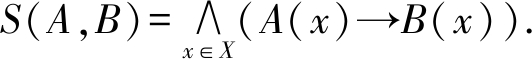1) aS(A,B)=S(aA,B)=S(A,aB)；

2) S(AB,C)=S(A,C)∧S(B,C)；

3) S(A,BC)=S(A,B)∧S(A,C)；

4) S(A,B)⊗S(B,C)≤S(A,C).

## 2 含参模糊决策蕴涵的语义、语构及其性质

1) 语义方面的研究包括：①含参模糊决策蕴涵的合理性，即含参模糊决策蕴涵是否合法；②含参模糊决策蕴涵的无冗余性，即含参模糊决策蕴涵能否被其他含参模糊决策蕴涵导出；③含参模糊决策蕴涵的完备性，即含参模糊决策蕴涵是否包含完整的信息.

2) 语构方面的研究包括：①推理规则的合理性，即含参模糊决策蕴涵能否使用推理规则得出；②推理规则的完备性，即是否能用这些推理规则推出所有可能的含参模糊决策蕴涵.

### 2.1 含参模糊决策蕴涵的语义特征

AB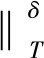=(δ1S(A,TC))*→(δ2
S(B,TD))=S(δ1A,TC)*S(δ2B,TD)，

AB={0.5/s,0.5/l}⟹{0.5/f,0.5/n}，
T={0.75/s,0/l,0.25/f,0.5/n}，
TC={0.75/s,0/l}，
TD={0.25/f,0.5/n}，

AB=(0.5→((0.5→0.75)∧
(0.5→0)))*→(0.5→((0.5→0.25)∧
(0.5→0.5))=1.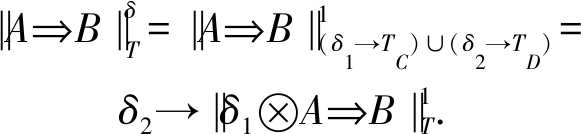AB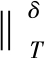=∨{cL|‖AcB=1}.

AB=∧{‖AB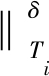|TiT}.

Modδ(L)={TLC×LD|L(AB)≤
AB}，

AB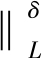=‖AB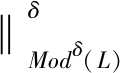ABL导出的程度等于L的全部模型满足AB的程度.

L={({0.5/s,0.25/l}⟹{0.25/f,0.5/n})/0.75,
({0/s,0.5/l}⟹{0.5/f,0/n})/0.75}，

δ1=δ2=0.5.对于T={0.5/s,0/l,0/f,0.5/n}，使用ukasiewicz伴随对和Identity语气真值算子计算可得

‖{0.5/s,0.25/l}⟹{0.25/f,0.5/n}=1≥0.75，
‖{0/s,0.5/l}⟹{0.5/f,0/n}=1≥0.75.

1) Modδ(L)={T|(δ1TC)∪(δ2TD)∈Mod1(L)}；

2)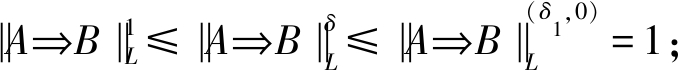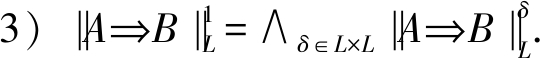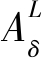=∪{L(A1B1)⊗S(δ1A1,A)*B1|
A1LC,B1LD}.

L={(A1B1)/0.75,(A2B2)/0.75}=
{({0.5/s,0.25/l}⟹{0.25/f,0.5/n})/0.75,
({0/s,0.5/l}⟹{0.5/f,0/n})/0.75}，

L(A1B1)⊗S(δ1A1,A)*B1=
0.75⊗1⊗B1={0/f,0.25/n}，
L(A2B2)⊗S(δ1A2,A)*B2={0/f,0/n}.

L(A1B1)⊗S(δ1A1,A)*B1=
0⊗S(δ1A1,A)*B1={0/f,0/n}，

c=L(A1B1)⊗S(δ1A1,A)*

(cB1(u)⊗δ2)→(cB1(u)⊗δ2)=1.

(cB1δ2)→(cB1δ2)=
cS(δ2B1,δ2cB1)=1，

L(A1B1)⊗S(δ1A1,A)*
S(δ2B1,δ2L(A1B1)⊗
S(δ1A1,A)*B1).

δ2δ2L(A1B1)⊗
S(δ1A1,A)*B1

L(A1B1)⊗S(δ1A1,A)*
S(δ2B1,δ2)，

L(A1B1)≤S(δ1A1,A)*
S(δ2B1,δ2)，

T0=A∪(δ2)={0.5/s,0/l,0/f,0/n}，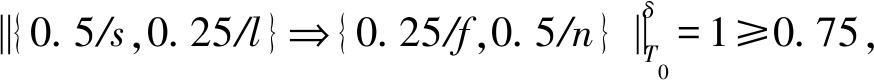‖{0/s,0.5/l}⟹{0.5/f,0/n}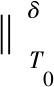=1≥0.75，

L(A1B1)≤‖A1B1=
S(δ1A1,TC)*S(δ2B1,TD)，

L(A1B1)⊗S(δ1A1,TC)*
S(δ2B1,TD)⟺L(A1B1)⊗
S(δ1A1,TC)*
S(δ2B1,TD)=1.

S(δ1A1,δ1A)*
S(δ1A,TC)*S(δ1A1,TC)*

L(A1B1)⊗S(δ1A1,δ1A)*S(δ1
A,TC)*L(A1B1)⊗S(δ1A1,TD)*

L(A1B1)⊗S(δ1A1,δ1A)*
S(δ1A,TC)*S(δ2B1,TD)≥
L(A1B1)⊗S(δ1A1,TC)*
S(δ2B1,TD)=1，

L(A1B1)⊗S(δ1A1,δ1A)*
S(δ1A,TC)*S(δ2B1,TD)，

S(δ1A,TC)*L(A1B1)⊗
S(δ1A1,δ1A)*
S(B1,δ2TD)=
S(δ2L(A1B1)⊗
S(δ1A1,δ1A)*B1,TD)，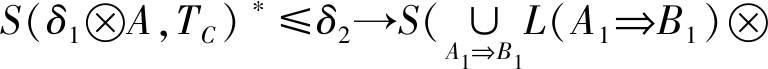S(δ1A1,δ1A)*B1,TD)，S(δ1A1,δ1A)*B1,TD)=
S(δ2⊗(δ1A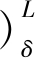,TD)，

S(δ1A,TC)*
S(δ2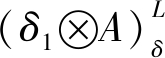,TD)=1，

A=1.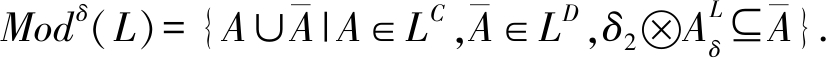Modδ(L)⊇{A∪(δ2)|ALC}，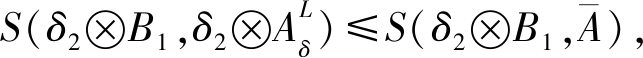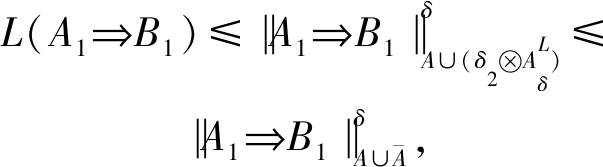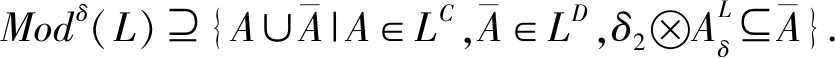L(A1B1)≤S(δ1A1,TC)*
S(δ2B1,TD)⟺L(A1B1)⊗
S(δ1A1,TC)*S(δ2B1,TD)⟺
L(A1B1)⊗S(δ1A1,TC)*
δ2B1(d)→TD(d),∀dD
L(A1B1)⊗S(δ1A1,TC)*
δ2B1(d)≤TD(d),∀dD
L(A1B1)⊗S(δ1A1,TC)*
δ2B1TD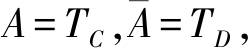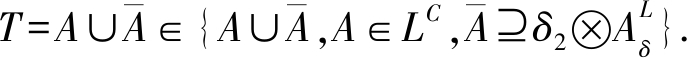Table 1 Closure and Models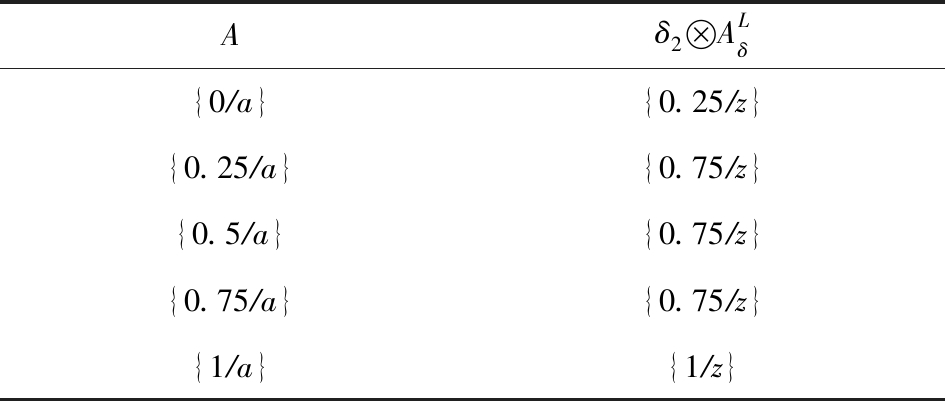Aδ2 ALδ{0∕a}{0.25∕z}{0.25∕a}{0.75∕z}{0.5∕a}{0.75∕z}{0.75∕a}{0.75∕z}{1∕a}{1∕z}

Modδ(L)={{0/a,0.25/z},{0/a,0.5/z},
{0/a,0.75/z},{0/a,1.0/z},{0.25/a,0.75/z},
{0.25/a,1/z},{0.5/a,0.75/z},{0.5/a,1/z},
{0.75/a,0.75/z},{0.75/a,1/z},{1/a,1/z}}.

T={0.5/a,0/z}，计算有‖AB=0.2<1，所以T不是该决策蕴涵集的模型.

AB=S(δ2B,δ2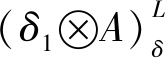).

AB=∧TModδ(L)AB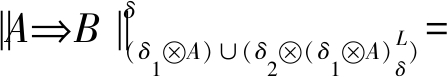S(δ2B,δ2⊗(δ1A).

A=1，

S(δ1A,TC)*S(δ2,TD)=1，

S(δ1A,TC)*S(δ2,TD)，

S(δ2B,δ2⊗(δ1A)⊗
S(δ2⊗(δ1A),TD)≤S(δ2B,TD)，

S(δ2B,δ2⊗(δ1A)⊗
S(δ1A,TC)*S(δ2B,TD)，

S(δ2B,δ2⊗(δ1A)≤
S(δ1A,TC)*S(δ2B,TD)，

S(δ2B,δ2)≤‖AB.T的任意性有

S(δ2B,δ2⊗(δ1A)≤
TModδ(L)AB=‖AB.

### 2.2 含参模糊决策蕴涵的语构特征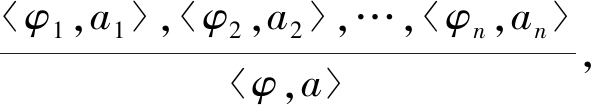1) 含参模糊变换推理规则：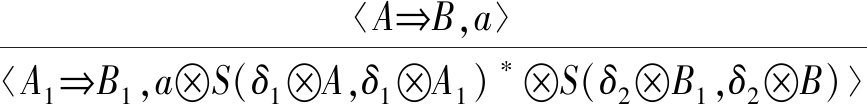.

2) 含参模糊扩增推理规则：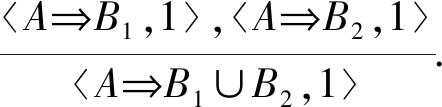3) 含参模糊转换推理规则：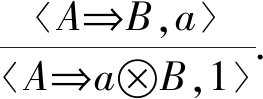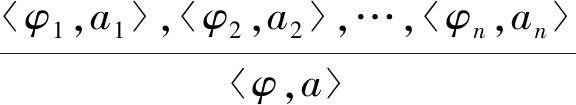Mod({a11,a22,…,ann})=
Mod({a11,a22,…,ann,a/φ}).

aS(δ1A,δ1A1)*S(δ2B1,δ2B)≤
A1B1=S(δ1A1,TC)*
S(δ2B1,TD)，

aS(δ1A,δ1A1)*S(δ2B1,δ2B)⊗
S(δ1A1,TC)*S(δ2B1,TD)，

ABS(δ1A,δ1A1)*S(δ2B1,
δ2B)⊗S(δ1A1,TC)*S(δ2B1,TD)，

S(δ2B1,δ2B)⊗S(δ2B,TD)≤
S(δ2B1,TD)，

(1)

(S(δ1A,TC)*S(δ2B,TD))⊗
S(δ1A,TC)*S(δ2B,TD)，

(2)

(S(δ1A,TC)*S(δ2B,TD))⊗
S(δ1A,TC)*S(δ2B1,δ2B)≤
S(δ2B,TD)⊗S(δ2B1,δ2B)≤
S(δ2B1,TD)，

ABS(δ1A,TC)*
S(δ2B1,δ2B)≤S(δ2B1,TD)，

S(δ1A,δ1A1)*S(δ1A1,TC)*
S(δ1A,TC)*

ABS(δ1A,δ1A1)*
S(δ1A1,TC)*S(δ2B1,δ2B)≤
S(δ2B1,TD)，

ABS(δ1A,δ1A1)*S(δ2B1,
δ2B)≤S(δ1A1,TC)*S(δ2B1,TD)，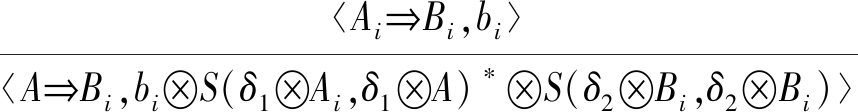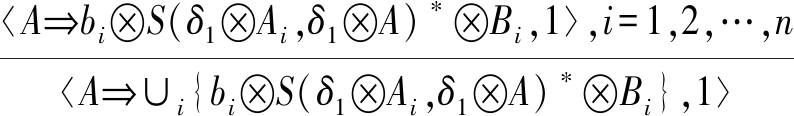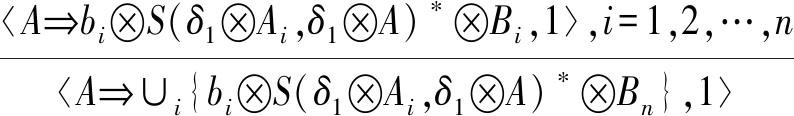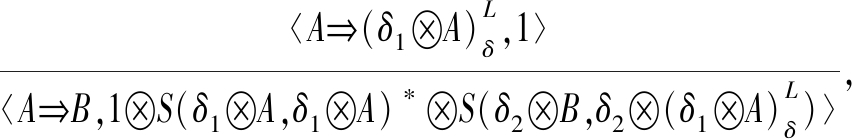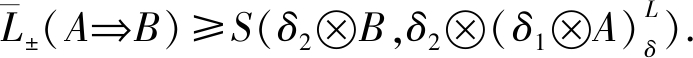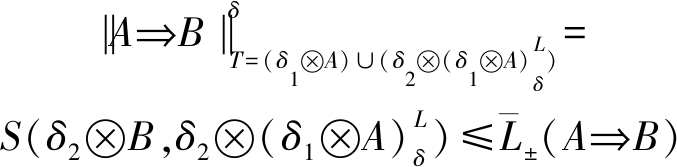## 3 结 论

Ganter B, Wille R. Formal Concept Analysis-Mathematical Foundations[M]. Berlin: Springer, 1999

Xu Weihua, Li Wentao. Granular computing approach to two-way learning based on formal concept analysis in fuzzy datasets[J]. IEEE Transactions on Cybernetics, 2016, 46(2): 366-379

Xu Jiaqing, Peng Xin, Zhao Wenyun. Program clustering for comprehension based on fuzzy formal concept analysis[J]. Journal of Computer Research and Development, 2009, 46(9): 1556-1566 (in Chinese)(许佳卿, 彭鑫, 赵文耘.一种基于模糊形式概念分析的程序聚类方法[J]. 计算机研究与发展, 2009, 46(9): 1556-1566)

Nicolas J, François K, Amedeo N. Analysis of social communities with iceberg and stability-based concept lattices[G] //LNCS 4933:Proc of the 6th Int Conf on Formal Concept Analysis. Berlin: Springer, 2008: 258-272

Xu Weihua, Pang Jinzhong, Luo Shuqun. A novel cognitive system model and approach to transformation of information granules[J]. International Journal of Approximate Reasoning, 2014, 55(3): 853-866

Li Jinhai, Mei Changlin, Xu Weihua, et al. Concept learning via granular computing: A cognitive viewpoint[J]. Information Sciences, 2015, 298(12): 447-467

Li Jinhai, Li Yufei, Mi Yunlong, et al. Meso-granularity labeled method for multi-granularity formal concept analysis[J]. Journal of Computer Research and Development, 2020, 57(2): 447-458 (in Chinese)(李金海, 李玉斐, 米允龙, 等. 多粒度形式概念分析的介粒度标记方法[J].计算机研究与发展, 2020, 57(2): 447-458)

Zhang Wenxiu, Wei Lin, Qi Jianjun. Attribute reduction theory and approach to concept lattice[J]. Science in China: Series E Information Sciences, 2005, 35(6): 628-639

Burusco A, Fuentes-Gonzáles R. The study of the L-fuzzy concept lattice[J]. Mathware and Soft Computing, 1970, 1(3): 209-218

Burusco A, Fuentes-González R. Construction of the L-fuzzy concept lattice[J]. Fuzzy Sets and Systems, 1998, 97(1): 109-114

Bělohlávek R. Fuzzy Relational Systems: Foundations and Principles[M]. Berlin: Springer, 2002

Bělohlávek R, Tatana F, Vychodil V. Galois connections with hedges[C] //Proc of the 11th Int Fuzzy Systems Association World Congress. Berlin: Springer, 2005: 1250-1255

Bělohlávek R, Vychodil V. Fuzzy concept lattices constrained by hedges[J]. Journal of Advanced Computational Intelligence and Intelligent Informatics, 2007, 11(6): 536-545

Bělohlávek R, Outrata J, Vychodil V. Thresholds and shifted attributes in formal concept analysis of data with fuzzy attributes[C] //Proc of the 6th Int Conf on Computational Science. Berlin: Springer, 2006: 117-130

Zhang Wenxiu, Ma Jianmin, Fan Shiqing. Variable threshold concept lattices[J]. Information Sciences, 2007, 177(22): 4883-4892

Qu Kaishe, Zhai Yanhui. Generating complete set of implications for formal contexts[J]. Knowledge-Based Systems, 2008, 21(5): 429-433

Qu Kaishe, Zhai Yanhui, Zhao Yanmeng. Study of decision implications based on formal concept analysis[J]. Journal of Systems Science and Information, 2006, 4(3): 533-542

Zhai Yanhui, Li Deyu, Qu Kaishe. Decision implications: A logical point of view[J]. International Journal of Machine Learning and Cybernetics, 2014, 5(4): 509-516

Zhai Yanhui, Li Deyu, Qu Kaishe. Decision implication canonical basisi[J]. Acta Electronica Sinica, 2015, 43(1): 18-23 (in Chinese)(翟岩慧, 李德玉, 曲开社. 决策蕴涵规范基[J]. 电子学报, 2015, 43(1): 18-23)

Zhang Shaoxia, Li Deyu, Zhai Yanhui, et al. A comparative study of decision implication, concept rule and granular rule[J]. Information Sciences, 2020, 508(8): 33-49

Wu Weizhi, Leung Yee, Mi Jushen. Granular computing and knowledge reduction in formal contexts[J]. IEEE Transactions on Knowledge & Data Engineering, 2009, 21(10): 1461-1474

Li Jinhai, Mei Changlin, Kumar C A, et al. On rule acquisition in decision formal contexts[J]. International Journal of Machine Learning and Cybernetics, 2013, 4(6): 721-731

Zhai Yanhui, Li Deyu, Zhang Jin. Variable decision knowledge representation: A logical description[J]. Journal of Computational Science, 2017, 25(5): 161-169

Zhai Yanhui, Li Deyu, Qu Kaishe. Fuzzy decision implications[J]. Knowledge-Based Systems, 2013, 37(8): 230-236

Zhai Yanhui, Li Deyu, Qu Kaishe. Fuzzy decision implication canonical basis[J]. International Journal of Machine Learning and Cybernetics, 2018, 9(11): 1909-1917

Pavelka J. On fuzzy logic I: Many-valued rules of inference[J]. Mathematical Logic Quarterly, 1979, 25(3-6): 45-52

# Parameterized Fuzzy Decision Implication

Wang Qi1, Li Deyu1,2, Zhai Yanhui1,2, and Zhang Shaoxia1

1(School of Computer and Information Technology, Shanxi University, Taiyuan 030006)

2(Key Laboratory of Computational Intelligence and Chinese Information Processing(Shanxi University), Ministry of Education, Taiyuan 030006)

Abstract Intelligent decision making is an important part of artificial intelligence. In formal concept analysis, decision is represented by decision implication in decision context, while fuzzy decision implication is based on fuzzy decision context, whose premise and conclusion are condition attributes and decision attributes respectively. Fuzzy decision implication exhibits a wider application significance, because it can avoid the fuzzy attribute implications that occur between condition attributes and between decision attributes. Deterministic, unadjustable knowledge acquisition is poorly adaptive to practical applications. Therefore, we need to expand unadjustable knowledge discovery methods to parameterized adjustable knowledge discovery methods. As two kinds of parameterized strategies, hedges and thresholds play an important role in the research of formal concept analysis with fuzzy attributes. Existing fuzzy decision implication models only take into account the hedge operator, which is poor in tunability, and the parameterized strategy that considers the threshold is less studied. Thus, in our paper, complete residual lattice is used as the reference frame, and the two parameterized strategies of hedge and threshold are introduced into fuzzy decision implication. We study the semantic aspect and prove some basic properties. Then we put forward three inference rules for parameterized fuzzy decision implication based knowledge reasoning and show their rationality and completeness. According to the results obtained, in real life, users may choose appropriate hedge and threshold to acquire knowledge, thus enhancing the tunability and application value of fuzzy decision implication.

Key words formal concept analysis; parameterized fuzzy decision implication; parameterize; fuzzy logic; fuzzy decision implication

DOI:10.7544/issn1000-1239.20210539

This work was supported by the National Natural Science Foundation of China (62072294, 61972238, 61806116) and the Key Research and Development Program of Shanxi Province (International Cooperation) (201803D421024, 201903D421041).Wang Qi, born in 1997. Master. His main research interests include data mining and intelligent decision.Li Deyu, born in 1965. PhD, professor, PhD supervisor. Senior member of CCF. His main research interests include rough set, multi-label learning.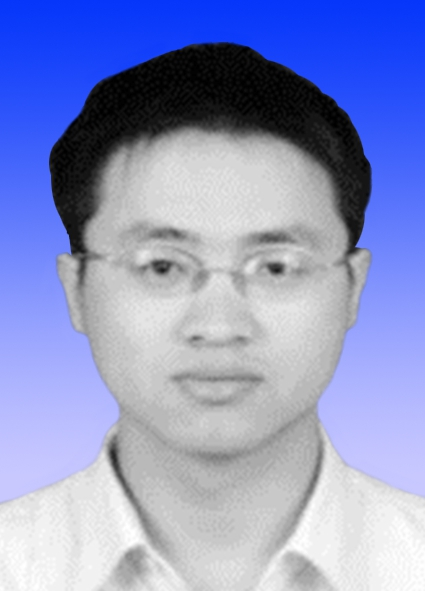Zhai Yanhui, born in 1981. PhD, associate professor, PhD supervisor. Member of CCF. His main research interests include concept lattice and rough set.Zhang Shaoxia, born in 1991. PhD. Her main research interest is concept lattice.